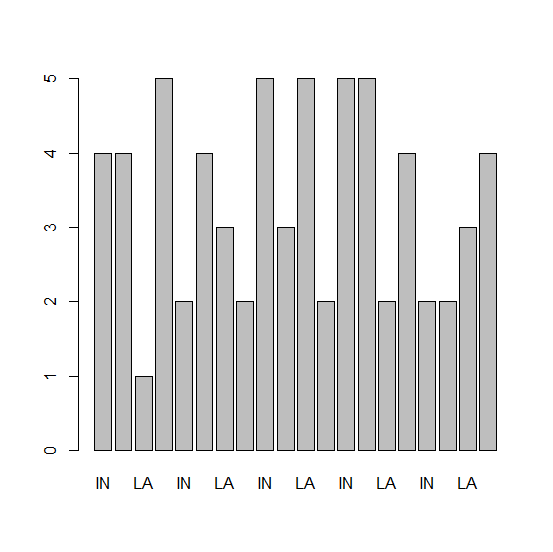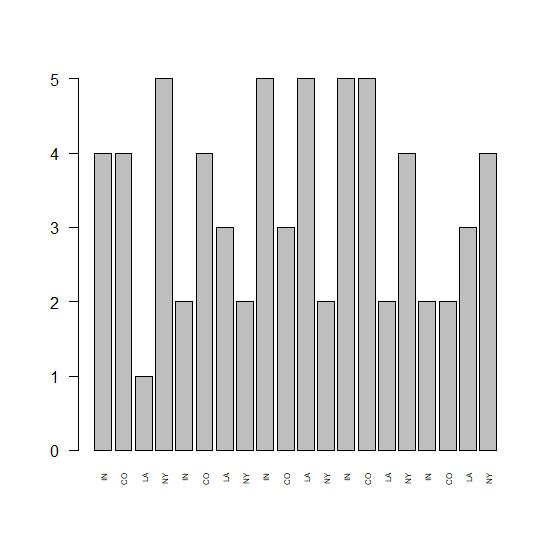# How to show all X-axis labels in a bar graph created by using barplot function in R?

R ProgrammingServer Side ProgrammingProgramming

In base R, the barplot function easily creates a barplot but if the number of bars is large or we can say that if the categories we have for X-axis are large then some of the X-axis labels are not shown in the plot. Therefore, if we want them in the plot then we need to use las and cex.names.

## Example

Consider the below data and bar graph −

Live Demo

> x<-sample(1:5,20,replace=TRUE)
> names(x)<-rep(c("IN","CO","LA","NY"),times=5)
> barplot(x)

## OutputShowing all the X-axis labels −

> barplot(x,las=2,cex.names=0.5)

## Output